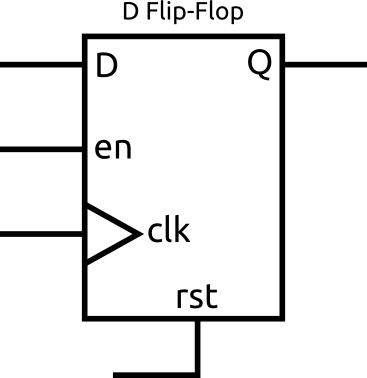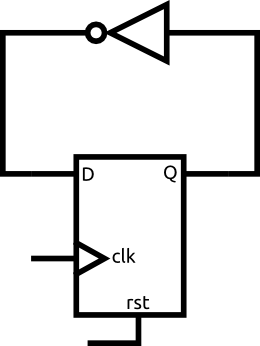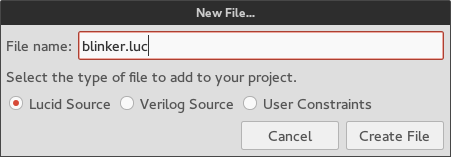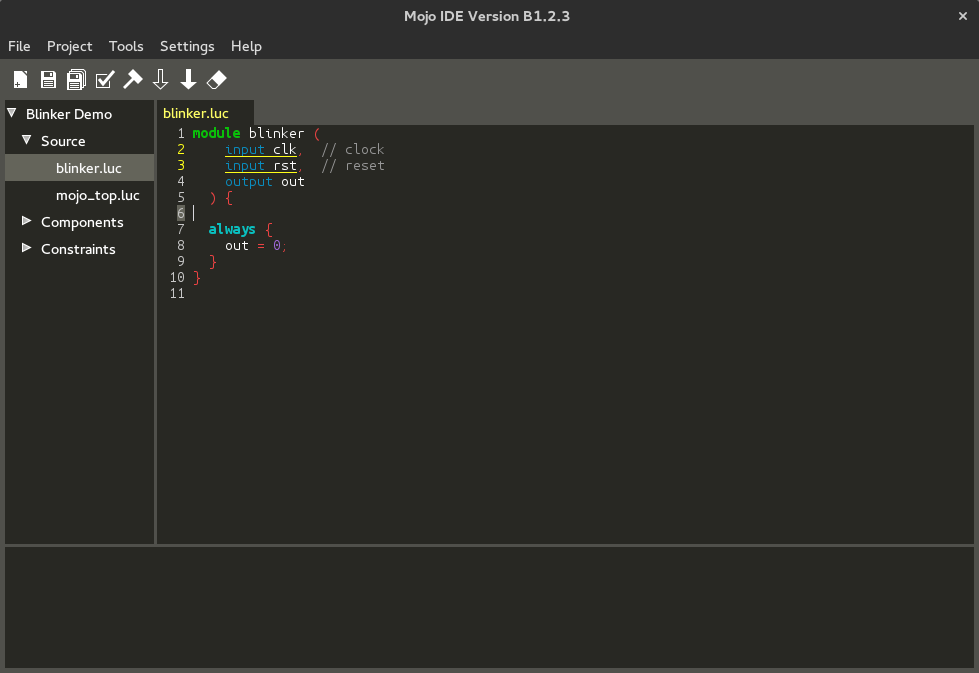# Synchronous Logic

Synchronous logic is a fundamental building block for just about any digital design. It allows you to create much more complex systems that accomplish something over a series of steps. It also gives you the ability to save states or other information. In this tutorial you will be introduced to the D flip flop and how you can use these to make an LED blink.

## The Problem

In our last tutorial we simply wired a LED to a button. Whenever you pressed the button the LED would turn on. Our design had no concept of time. The goal for this project is to blink an LED. That means we have to create a circuit that can turn itself on and off automatically after a regular interval of time has passed. For this we need the flip flop.

## Clocks

Before I can explain what exactly a flip flop does you need to understand what a clock is.

A clock is just a signal that toggles between 0 and 1 over and over again. It looks something like this.The important thing is the rate at which it toggles. The clock on the Au and Cu is a 100MHz clock (the Mojo has a 50MHz clock). That means that it toggles 100 million times per second!

The clock has two edges, the rising edge and the falling edge. I drew the rising edges with little arrows. The rising edge is usually the important one.

## D Flip-Flops

This is one of the most important circuit elements you will be using. Lucky for you, it is also fairly straightforward how they work. Take a look at the symbol for the flip flop below.This image shows all the signals that a flip flop could have, but in practice, the only required signals are D, Q, and clk. For now, forget about rst and en.

So what exactly does this thing do? All it does is copy the signal at D to Q whenever there is a rising edge on the clk input. That means Q will keep it's value between rising edges of the clock. Since the flip flop remembers what the input was at D, it is actually one of the most basic memory elements.

## Loops

Lets take a look at the following example.What will this circuit do? If the input to the gate is 1, then it's output is 0. However, the output is the input so the output must be 1, but then the input is 1 so the output must be 0? If we assume that the signal can only be 0 or 1 it seems like it would toggle between 0 and 1 infinitely fast. In practice, remember that signals are actually represented by voltages, it may oscillate or it may settle to somewhere in the middle. This is, of course, something we don't want. Designing combinational circuits with feedback can be very tricky to make sure something like this doesn't happen and that the circuit will work how you expect it to. That is why we don't! Instead, we use a circuit like this one.What will this circuit do? Well, for now lets just assume that Q is 0. That means that D is 1 (because it went through the not gate). On the next rising edge of the clock Q will copy what D is, so Q becomes 1. Once Q becomes 1, D becomes 0. You can follow the pattern to realize that every time there is a rising edge on the clock the output of the flip flop toggles.

What about the initial condition though? If we just built this circuit how do we know if Q is 0 or 1? The truth is that we don't and in some cases it may be 1 while others it may be 0. That is where the rst signal comes in. This signal is used to reset the flip flop to a known state. In FPGAs this signal is generally very flexible and allows you to reset the flip flop to a 1 or 0 when the signal is high or low (your choice, not both). In Lucid, dffs use active high resets. That means when the rst signal is 1, the flip flop is held in reset.

There are cases where you don't care what the initial value of the flip flop is, in those cases you don't need to, and shouldn't, use a reset.

Since the only signal left is en, I'll cover it now just for completeness. There are times when you want the flip flop to ignore the rising edges on the clock and to preserve the contents of Q. That is when you use the enable signal. When the en signal is 1, the flip flop operates normally. When it is 0 the contents of Q won't change on the rising edges of the clock. If you see a flip flop without an en signal it is just assumed that the flip flop is always enabled.

In Lucid, dffs don't have an explicit en signal, but rather will retain their contents if you don't write something new to it.

## Creating the Module

Open Alchitry Labs and create a new project. I'm going to call mine BlinkerDemo.

With the new project open, click the New File icon (the left most icon in the toolbar) and create a Lucid source file called blinker.luc.Click Create File to create the file.

The file should now be under Source in the project tree and it should automatically open.Edit the module so that it looks like the following.

```module blinker (
input clk,    // clock
input rst,    // reset
output blink  // output to LED
) {

dff counter(.clk(clk), .rst(rst));

always {
counter.d = counter.q + 1;
}
}
```

Let's go over what changes were made. First, I simply renamed out to blink to better reflect what our module does.

Line 7 has the declaration of the flip-flop. Lucid has a type dff for creating flip-flops. The flip-flop I created is called counter and it's 25 bits wide.

I then connected the clk signal of our module to the clk input of the flip-flop. I did the same with the rst signal. The syntax for connecting these signals is .module_input(signal) where module_input is the name of the input on the module and signal is the signal to connect to it. In this case the module input and signal names are the same.

In the always block, line 10 simply connects our output, blink, to the most significant bit of counter.q. When you are working with flip-flops, FSMs, or modules, you use the dot syntax to specify which signal you want. In this case, we need to read the q output of the dff so we use counter.q.

The next line connects the input, d, of the dff to its output, q, plus one. This means that every time clk goes high, counter.q will increase by 1.

When declaring a dff or fsm, you must connect its clk input and optionally connect rst. These can't be connected later in an always block.

## Reset

Notice we connect the rst signal to the counter. What does this do? Whenever the rst signal goes high, the value of counter.q becomes 0. This is also the value that the counter is initialized to when the FPGA first starts.

If we wanted the counter to initialize and reset to a different value, we can specify the value using the dff parameter #INIT.

`dff counter(#INIT(100), .clk(clk), .rst(rst));`

The counter will now start with a value of 100 and reset to 100. Zero is the default value if none is specified.

Notice that parameters are specified with #NAME instead of .name. Parameters are always all capitalized.

If you don't need to reset a dff for some reason, you can simply not connect anything to the rst input and it won't have a reset. This is recommended if you don't need a reset since it doesn't force the tools to route the reset signal to the flip-flop.

The dff and fsm (covered later) types are special in that the rst input is optional. All other inputs and all inputs to modules are required.

## The Counter

Here is what the counter circuit looks like. Keep in mind that there is actually 25 flip-flops (but only one +1 circuit) and the connections are actually 25 wires, or bits, wide. When many flip-flops are used to store a single multi-bit value they are commonly drawn as a single flip-flop.Let's look at what this module will actually do. Right after the rst signal goes low, counter.q will be 0. That means that counter.d will be 1, since our combinational block assigns it counter.q plus 1.

At the next positive edge of clk, counter.q will be assigned the value of counter.d, or 1. Once counter.q is 1, counter.d must be 2. You should be able to see what will continue to happen. Each clock cycle counter.q will increase by 1. But what happens when counter.q is 25b1111111111111111111111111 (the max value)?

Since we are adding a 1 bit number to a 25 bit number, the result can be up to 26 bits, but since we are storing it into a 25 bit dff we lose the last bit. That means that when counter.q is the max value, counter.d is 0 and the process starts all over again.

Our counter will continuously count from 0 to 33554431 (2^25 - 1).

How do we make an LED blink from this counter? It's simple once you realize that for half the time the most significant bit is 1 and the other half of the time it's 0. This is because if counter.q is less than 16777216 (2^24), then the 24th but must be 0 and when it is equal or greater, the MSB must be 1. That means we can just connect the LED to the most significant bit of our counter. If you need to convince yourself this is true try writing out the binary values from 0-7 and look at how the MSB (most significant bit) changes.

How fast will the LED blink though? We know that the clock frequency is 100MHz, or 100,000,000 clock cycles per second on the Au and Cu. Since our counter takes 2^25 cycles to overflow we can calculate the blinker time, 2^25 / 100,000,000 or about 0.34 seconds (0.67 seconds on the Mojo). The LED will turn on and off every 0.34 seconds. If you wanted to make that time longer, you can just make the counter 26 bits long and the time will double to 0.67 seconds. If you wanted to make it blink faster you can make the counter shorter.

## Module connections

In Lucid, there are three ways to specify an input into a module, dff, or fsm (from now on I'll just call them all modules). The first way is how we did it in this example.

```dff counter(.clk(clk), .rst(rst));
```

Here we specify the connections in a set of parentheses directly after the name. These connections are only applied to this single module. However, we can use the next method to make the same connections to many modules.

```.clk(clk), .rst(rst) {
dff counter1;
dff counter2;
dff counter3;
}
```

In this example, we connect the clk and rst inputs of all the modules contained in the curly braces. In this case, they contain three dffs. This is convenient since most modules will require a clock and reset signal.

You can also nest this method.

```.clk(clk) {
.rst(rst) {
dff counter1;
dff counter2;
}
dff counter3;
}
```

Here only counter1 and counter2 are connected to rst.

You can also mix this method with the first.

```.clk(clk) {
dff counter1(.rst(rst));
dff counter2;
dff counter3;
}
```

Here only counter1 is connected to rst.

Finally, the last way to specify an input is simply by not connecting it when declaring the module but rather inside an always block later. This is what we did with counter.d. Note that dffs and fsms require you to specify clk and rst when declaring them and they do not allow you to specify the d input then.

There is a difference when connecting an input at declaration time or in an always block. The difference only is noticeable for arrays. If you have an array of a module and you specify an input at declaration time, that input will be copied to each element in the array individually.

If you specify an input in an always block, you can specify the input to each element separately. In this example, the clk signal is one bit, but it is getting copied to 25 one bit flip-flops. However, the d input, which is also one bit, is packed into a 25 bit array to use in the always block. That way we can use the d input as if it is really one big flip-flop (which don’t actually exist).

## Instantiating a module

Now that we have a blinker module we need to add it to our top level module.

Open up the top file and make the edits so it looks the same as below.

```module au_top (
input clk,              // 100MHz clock
input rst_n,            // reset button (active low)
output led ,         // 8 user controllable LEDs
input usb_rx,           // USB->Serial input
output usb_tx           // USB->Serial output
) {

sig rst;                  // reset signal

.clk(clk) {
// The reset conditioner is used to synchronize the reset signal to the FPGA
// clock. This ensures the entire FPGA comes out of reset at the same time.
reset_conditioner reset_cond;

.rst(rst) {
}
}

always {
reset_cond.in = ~rst_n;    // input raw inverted reset signal
rst = reset_cond.out;      // conditioned reset

usb_tx = usb_rx;           // echo the serial data
}
}
```
```module cu_top (
input clk,              // 100MHz clock
input rst_n,            // reset button (active low)
output led ,         // 8 user controllable LEDs
input usb_rx,           // USB->Serial input
output usb_tx           // USB->Serial output
) {

sig rst;                  // reset signal

.clk(clk) {
// The reset conditioner is used to synchronize the reset signal to the FPGA
// clock. This ensures the entire FPGA comes out of reset at the same time.
reset_conditioner reset_cond;

.rst(rst) {
}
}

always {
reset_cond.in = ~rst_n;    // input raw inverted reset signal
rst = reset_cond.out;      // conditioned reset

usb_tx = usb_rx;           // echo the serial data
}
}
```
```module mojo_top (
input clk,              // 50MHz clock
input rst_n,            // reset button (active low)
output led ,         // 8 user controllable LEDs
input cclk,             // configuration clock, AVR ready when high
output spi_miso,        // AVR SPI MISO
input spi_ss,           // AVR SPI Slave Select
input spi_mosi,         // AVR SPI MOSI
input spi_sck,          // AVR SPI Clock
output spi_channel , // AVR general purpose pins (used by default to select ADC channel)
input avr_tx,           // AVR TX (FPGA RX)
output avr_rx,          // AVR RX (FPGA TX)
input avr_rx_busy       // AVR RX buffer full
) {

sig rst;                  // reset signal

.clk(clk) {
// The reset conditioner is used to synchronize the reset signal to the FPGA
// clock. This ensures the entire FPGA comes out of reset at the same time.
reset_conditioner reset_cond;

.rst(rst) {
}
}

always {
reset_cond.in = ~rst_n;    // input raw inverted reset signal
rst = reset_cond.out;      // conditioned reset

spi_miso = bz;             // not using SPI
spi_channel = bzzzz;       // not using flags
avr_rx = bz;               // not using serial port
}
}
```

In the nested .clk(clk) and .rst(rst) blocks, I instantiated the blinker module and named it myBlinker. Notice I'm using the batch way of connecting the clk and rst inputs this time. This is because you will likely want to add more modules to your top level module so it can nice to set it up beforehand.

In the always block, we connect the blink output of myBlinker to the eight LEDs using the duplication syntax covered in the previous tutorial.

You should now be able to build and load the project. All 8 LEDs should blink about two times per second.

## The Reset Conditioner

Now that we have actually used the reset signal for what it was intended for, we can talk about the reset_conditioner module. The signal rst_n comes from outside the FPGA. Signals from outside the FPGA are UNCLEAN! What I mean by this is that we don't know how external signals (especially from a button) will change in relation to the clock we are using. If the reset signal goes low really close to the rising edge of the clock, due to internal delays in the FPGA, some flip-flops may come out of reset before the rising edge while others could after. This means some flip-flops may stay reset for a cycle longer than others (NOT GOOD). Even worse, when signals change too close to a rising edge of a clock you run into metastability issues. This is covered later, but it basically means you aren't guaranteed the output of the flip-flop will be 1 or 0. It could be somewhere in between (0.5?) or even oscillate between values (BAD). This is where the reset conditioner comes in. It is a fairly simple circuit that synchronizes the reset signal to the FPGA's clock. This ensures that your entire design will come out of reset at once. If you want to read more than you'll ever want to know about resets, check out this paper from Xilinx.